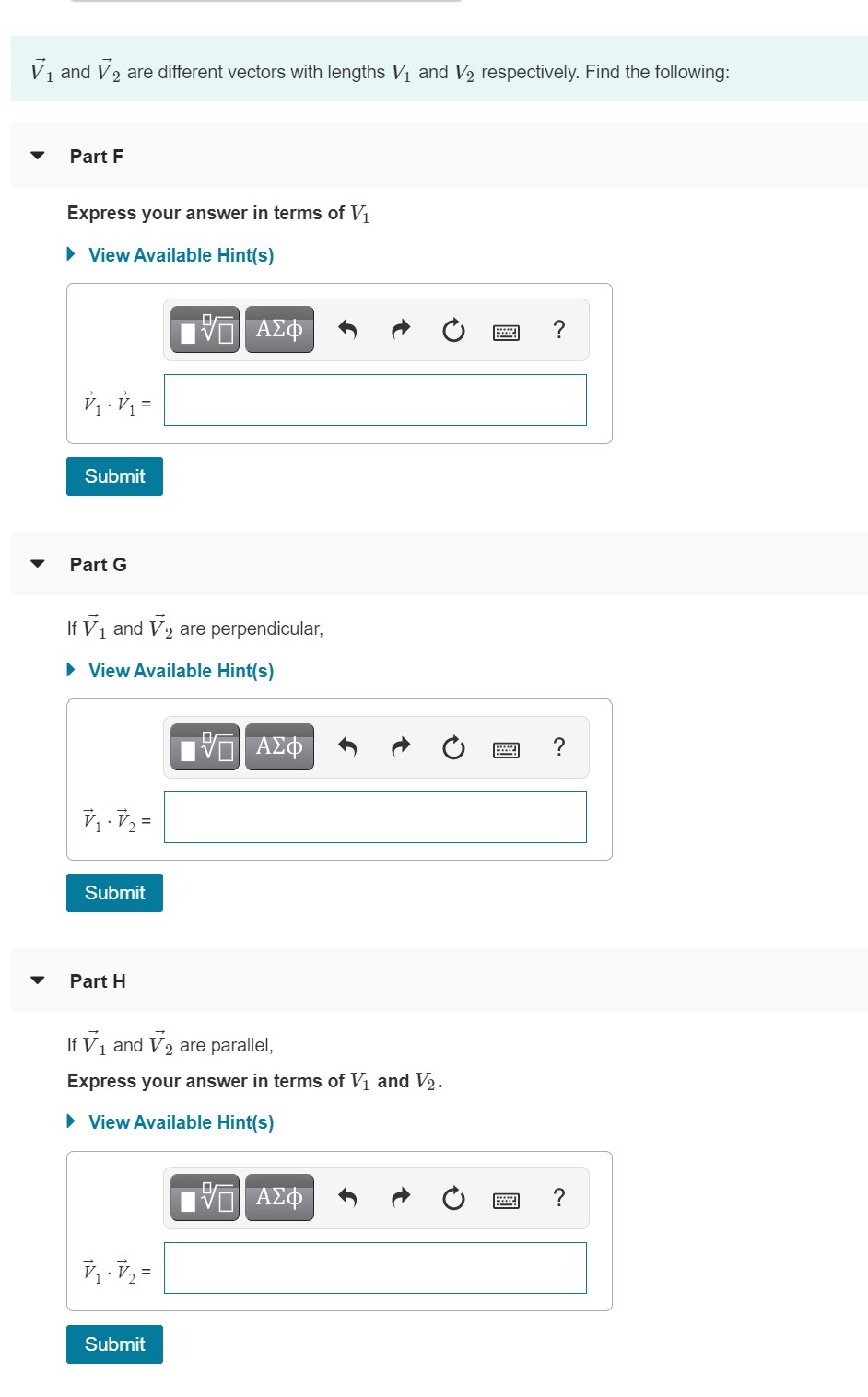# Question Solved1 AnswerLet vectors A⃗ =(2,1,−4)A→=(2,1,−4), B⃗ =(−3,0,1)B→=(−3,0,1), and C⃗ =(−1,−1,2)C→=(−1,−1,2). Calculate the following: Vi and V2 are different vectors with lengths Vị and V2 respectively. Find the following: Part F Express your answer in terms of Vi ► View Available Hint(s) ΟΙ ΑΣφ ? V.VN Submit Part G If V, and V2 are perpendicular, View Available Hint(s) ΟΙ ΑΣφ ? V.V2 = Submit Part 1 If V, and V2 are parallel, Express your answer in terms of Vi and V2. ► View Available Hint(s) VO AEO ? 7.72 = SubmitLet vectors A⃗ =(2,1,−4)A→=(2,1,−4), B⃗ =(−3,0,1)B→=(−3,0,1), and C⃗ =(−1,−1,2)C→=(−1,−1,2).
Calculate the following:Transcribed Image Text: Vi and V2 are different vectors with lengths Vị and V2 respectively. Find the following: Part F Express your answer in terms of Vi ► View Available Hint(s) ΟΙ ΑΣφ ? V.VN Submit Part G If V, and V2 are perpendicular, View Available Hint(s) ΟΙ ΑΣφ ? V.V2 = Submit Part 1 If V, and V2 are parallel, Express your answer in terms of Vi and V2. ► View Available Hint(s) VO AEO ? 7.72 = Submit
More
Transcribed Image Text: Vi and V2 are different vectors with lengths Vị and V2 respectively. Find the following: Part F Express your answer in terms of Vi ► View Available Hint(s) ΟΙ ΑΣφ ? V.VN Submit Part G If V, and V2 are perpendicular, View Available Hint(s) ΟΙ ΑΣφ ? V.V2 = Submit Part 1 If V, and V2 are parallel, Express your answer in terms of Vi and V2. ► View Available Hint(s) VO AEO ? 7.72 = Submit